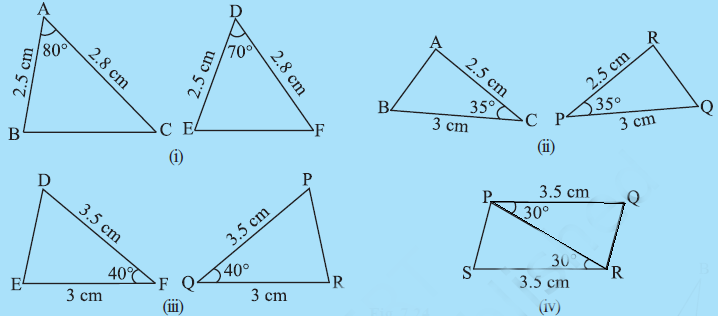Q

# In Fig 7.24, measures of some parts of the triangles are indicated. By applying SAS congruence rule, state the pairs of congruent triangles, if any, in each case In case of congruent triangles write them in symbolic form.

3. In Fig 7.24, measures of some parts of the triangles are indicated. By applying SAS congruence rule, state the pairs of congruent triangles, if any, in each case. In case of congruent triangles, write them in symbolic form.Views

i) in  and

AB = DE

AC = DF

Hence, they are not congruent.

ii) In  and

AC = RP = 2.5 cm

CB = PQ = 3 cm

Hence by SAS congruency rule, they are congruent.

.

iii) In  and

DF= PQ = 3.5 cm

FE= QR = 3 cm

Hence, by SAS congruency rule, they are congruent.

iv) In  and

QP = SR = 3.5 cm

PR = RP (Common side)

Hence, by SAS congruency rule, they are congruent.

.

Exams
Articles
Questions